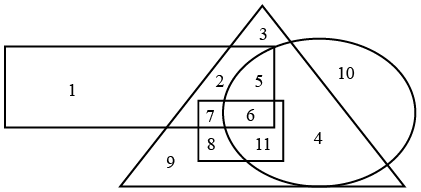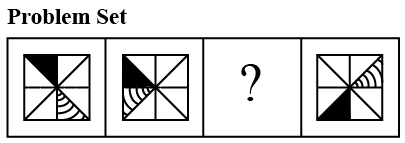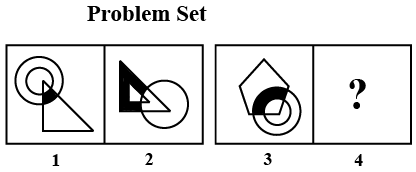Instructions

For the following questions answer them individually

Question 21

# A candidate obtains 60% marksin the first paper and 70% marks aggregate in two papers. What percentage marks he got in the second paper,if the fullmarks for two papersare in the ration of 2:3.Question 22

# What should be added to each of the numbers 12, 30, 40 and 86, so that they are in proportion:Question 23

#(1) The rectangle represents government employees.(2) The triangle represents urban people.(3) The circle represents graduates.(4) The square represents clerks.Which of the following Statement is true ?Question 24

# In the following question there is a set of four figures labeled 1, 2, 3 and 4 called the problem set followed by set of four other figures labeled a, b, c and d called the answer set. Figure 3 contains a Question Mark (?) .Select suitable figure from the answer set which will substitute the question mark (?) so that a series is formed by the figures 1, 2, 3, and 4 taken in order. The label of the selected figure is the answer.Question 25

# The following question consists of two sets of figures 1, 2,3 and 4 constitute the problem set while figures a, b, c and d constitute the answer set. There is a definite relationship between figures 1 and 2. Establish a similar relationship between figure 3 and 4 by choosing a suitable figure 4 from the answer set.Question 26

# An amount of money is distributed among P, Q and R in the ratio 7:3:11. If the total share of P & Q together is Rs.15,000/-, find out the difference between P and R's share.Question 27

# Different words are formed with the help of the letters of word ‘RELATION’. Find the number of words in which vowels always occupy the even places.Question 28

# Seven persons hand over the keys of their cars to a receptionist of a club. While leaving the club, they ask for the keys of their cars. All cars are of the same model of the same company. The receptionist hands over the keys to the members without knowing whose keys he is  handling over. How many times can two of them get the keys of their cars but remaining five can not?Question 29

# If P + Q’ means‘P is the son of Q’; ‘P - Q’ means‘P is the daughter of Q’; ‘P x Q’ means‘P is the brother of Q’: ‘P + Q’ means‘P is the sister of Q’. Then, how is A related with E in the given equation?$$A + B \times C \div D - E$$Question 30

# Fastest man in the recently concluded Beijing 2008 Olympics wasOR